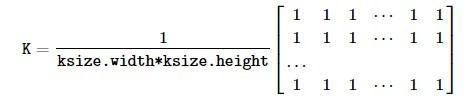# Python OpenCV | cv2.blur() method

OpenCV-Python is a library of Python bindings designed to solve computer vision problems. `cv2.blur()` method is used to blur an image using the normalized box filter. The function smooths an image using the kernel which is represented as:Syntax: cv2.blur(src, ksize[, dst[, anchor[, borderType]]])
Parameters:
src: It is the image whose is to be blurred.
ksize: A tuple representing the blurring kernel size.
dst: It is the output image of the same size and type as src.
anchor: It is a variable of type integer representing anchor point and it’s default value Point is (-1, -1) which means that the anchor is at the kernel center.
borderType: It depicts what kind of border to be added. It is defined by flags like cv2.BORDER_CONSTANT, cv2.BORDER_REFLECT, etc
Return Value: It returns an image.

Image used for all the below examples:Example #1:

 `# Python program to explain cv2.blur() method  ` ` `  `# importing cv2  ` `import` `cv2  ` ` `  `# path  ` `path ``=` `r``'C:\Users\Rajnish\Desktop\geeksforgeeks\geeks.png'` ` `  `# Reading an image in default mode  ` `image ``=` `cv2.imread(path)  ` ` `  `# Window name in which image is displayed  ` `window_name ``=` `'Image'` ` `  `# ksize ` `ksize ``=` `(``10``, ``10``) ` ` `  `# Using cv2.blur() method  ` `image ``=` `cv2.blur(image, ksize)  ` ` `  `# Displaying the image  ` `cv2.imshow(window_name, image)  `

Output:Example #2:

 `# Python program to explain cv2.blur() method  ` ` `  `# importing cv2  ` `import` `cv2  ` ` `  `# path  ` `path ``=` `r``'C:\Users\Rajnish\Desktop\geeksforgeeks\geeks.png'` ` `  `# Reading an image in default mode  ` `image ``=` `cv2.imread(path)  ` ` `  `# Window name in which image is displayed  ` `window_name ``=` `'Image'` ` `  `# ksize ` `ksize ``=` `(``30``, ``30``) ` ` `  `# Using cv2.blur() method  ` `image ``=` `cv2.blur(image, ksize, cv2.BORDER_DEFAULT)  ` ` `  `# Displaying the image  ` `cv2.imshow(window_name, image)  `

Output:My Personal Notes arrow_drop_upCheck out this Author's contributed articles.

If you like GeeksforGeeks and would like to contribute, you can also write an article using contribute.geeksforgeeks.org or mail your article to contribute@geeksforgeeks.org. See your article appearing on the GeeksforGeeks main page and help other Geeks.

Please Improve this article if you find anything incorrect by clicking on the "Improve Article" button below.

Article Tags :

Be the First to upvote.

Please write to us at contribute@geeksforgeeks.org to report any issue with the above content.